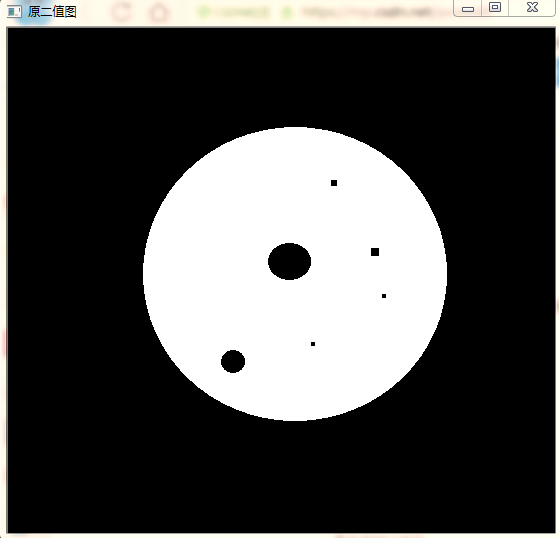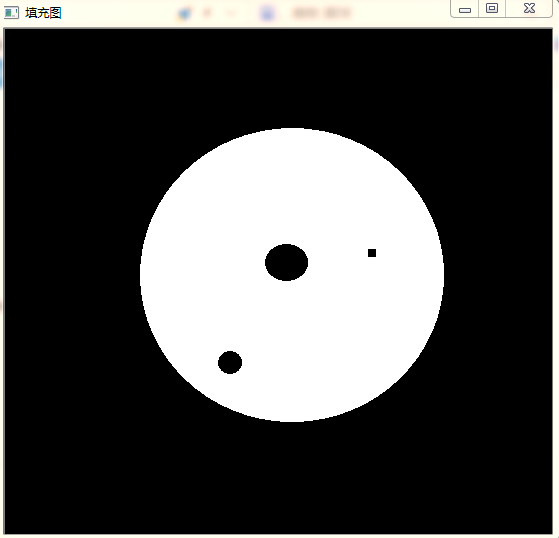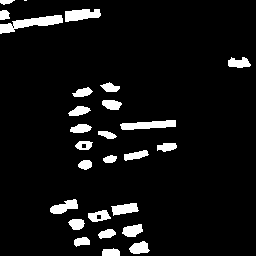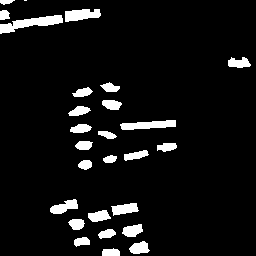2013-09-08 11:31:23 laviewpbt 阅读数 3414

2017-10-04 17:14:21 qq_30311601 阅读数 8717

（这里的二值为0和255）

1.确定二值图像像素[0,0]为初始种子点，这里认为[0,0]像素点为背景点，而非某个孔洞内部的点。

2.以种子点为起点，采用形态学膨胀算法对背景进行填充。膨胀到不能膨胀为止。膨胀运算采用四连通结构元。

3.背景填充结束后，对得到的二值图像取反得到新的二值图像，此时图像为全部孔洞的点集。

4.将第三步骤得到的二值图像与原二值图像相加及得到孔洞填充的结果。2018-04-22 11:45:37 jkjj2015 阅读数 7243

matlab中的imfill函数可以方便得实现二值图像的孔洞填充，而在opencv中并没有相同功能的函数。因此，在opencv的基础上编写实现孔洞填充的函数，并且能够设定阈值，对面积大于阈值的孔洞不进行填充。使用形态学重建的算法能够有效地实现孔洞填充，具体算法参照《数字图像处理》第三版9.5.9节，孔洞填充。

主要实现代码如下所示：其中imfill函数即为空洞填充的实现函数，第一个参数是二值图像（0~1），第二个参数是填充孔洞的阈值。若孔洞面积大于阈值则不填充，反之则填充。

```#include "iostream"
#include <opencv2\opencv.hpp>
using namespace std;
using namespace cv;
Mat inv_board(Mat src);
Mat inv_img(Mat src);
void delarea(Mat& bw, int max);
Mat imfill(Mat I, int max);
void main()
{
Mat I, src_gray, F_B, F_BI_C, temp, H, I_fill;
cvtColor(scr, src_gray, COLOR_BGR2GRAY);
threshold(src_gray, I, 0.1, 1,0);
I_fill = imfill(I,40);
imshow("原二值图", I * 255);
imshow("填充图", I_fill*255);
waitKey(0);
}

Mat imfill(Mat I,int max)
{
Mat  src_gray, F_B, F_BI_C, temp, H, I_fill;
I_fill = I.clone();
Mat F = inv_board(I);
Mat I_C = inv_img(I);
Mat element = getStructuringElement(0, Size(3, 3), Point(1, 1));
while (1)
{

dilate(F, F_B, element);
F_BI_C = F_B.mul(I_C);
temp = F_BI_C - F;
if (sum(temp) == Scalar(0))
break;
else
F = F_BI_C.clone();
}
H = inv_img(F_BI_C);
Mat H_IC = H.mul(I_C);
delarea(H_IC, max);
for (int i = 0; i < H_IC.rows; i++)
{
for (int j = 0; j < H_IC.cols; j++)
{
if (H_IC.at<uchar>(i, j) == 1)
I_fill.at<uchar>(i, j) = 1;
}
}
return I_fill;
}
Mat inv_board(Mat src)
{

int rows = src.rows;
int cols = src.cols;
Mat dst = Mat::zeros(rows, cols, CV_8UC1);
for (int i = 0; i < cols; i++)
{
dst.at<uchar>(0, i) = 1 - src.at<uchar>(0, i);
}
for (int i = 0; i < cols; i++)
{
dst.at<uchar>(rows-1, i) = 1 - src.at<uchar>(rows - 1, i);
}
for (int i = 1; i < rows-1; i++)
{
dst.at<uchar>(i, 0) = 1 - src.at<uchar>(i, 0);
}
for (int i = 1; i < rows - 1; i++)
{
dst.at<uchar>(i, cols-1) = 1 - src.at<uchar>(i, cols-1);
}
return dst;
}

Mat inv_img(Mat src)
{
int rows = src.rows;
int cols = src.cols;
Mat dst = src.clone();
for (int i = 0; i < rows; i++)
for (int j = 0; j < cols; j++)
dst.at<uchar>(i, j) = 1 - src.at<uchar>(i, j);
return dst;
}

void delarea(Mat& bw, int max )
{
Mat bw_copy = bw.clone();
int flag = 0;
Mat H_b, H_bw, temp;
Mat H = Mat::zeros(bw.size(), bw.type());
for (int i = 0; i < bw.rows; i++)
{
for (int j = 0; j < bw.cols; j++)
{
if (bw_copy.at<uchar>(i, j) == 1)
{
H.at<uchar>(i, j) = 1;
Mat element = getStructuringElement(0, Size(3, 3), Point(1, 1));
while (1)
{
dilate(H, H_b, element);
H_bw = H_b.mul(bw);
temp = H_bw - H;
if (sum(temp) == Scalar(0))
break;
else
H = H_bw.clone();
}
bw_copy = bw_copy - H_bw;
if (sum(H_bw).val > max)
{
bw = bw - H_bw;
}
H = Mat::zeros(bw.size(), bw.type());
}

}
}
}```2018-02-02 16:56:10 u014030821 阅读数 2406

# 第九章 形态学图像处理

## 一：腐蚀和膨胀

### 1.1 腐蚀

B对A的腐蚀是一个用z平移的B包含在A中的所有的点z的集合：### 1.2 膨胀## 二：开操作与闭操作## 三：击中或击不中变换## 四：一些基本的形态学算法

### 4.1 边界提取### 4.2 孔洞填充### 4.3 连通分量的提取### 4.4 凸壳### 4.5 细化### 4.6 粗化### 4.7 骨架### 4.8 裁剪### 4.9 形态学重建

#### 4.9.1 测地膨胀和腐蚀#### 4.9.2 用膨胀和腐蚀的形态学重建## 五：灰度级形态学

### 5.1 腐蚀和膨胀### 5.2 开操作和闭操作### 5.3 一些基本的灰度级形态学算法

#### 5.3.1 形态学平滑#### 5.3.2 形态学梯度#### 5.3.3 顶帽变换和底帽变换#### 5.3.4 粒度测定#### 5.3.5 纹理分割### 5.4 灰度级形态学重建2019-04-23 10:31:08 weixin_42181588 阅读数 840``````import cv2;
import numpy as np;

'''

'''
def FillHole(imgPath,SavePath):

# 复制 im_in 图像
im_floodfill = im_in.copy()

h, w = im_in.shape[:2]

# floodFill函数中的seedPoint必须是背景
isbreak = False
for i in range(im_floodfill.shape):
for j in range(im_floodfill.shape):
if(im_floodfill[i][j]==0):
seedPoint=(i,j)
isbreak = True
break
if(isbreak):
break
# 得到im_floodfill

# 得到im_floodfill的逆im_floodfill_inv
im_floodfill_inv = cv2.bitwise_not(im_floodfill)
# 把im_in、im_floodfill_inv这两幅图像结合起来得到前景
im_out = im_in | im_floodfill_inv

# 保存结果
cv2.imwrite(SavePath, im_out)

``````